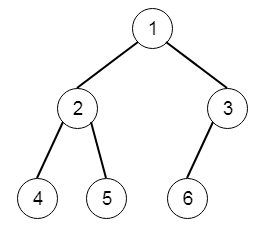# Check Completeness of a Binary Tree in C++

C++Server Side ProgrammingProgramming

Suppose we have a binary tree. We have to check whether the tree is a complete binary tree or not. A complete binary tree of level n, has n-1 complete levels, and all nodes at level n, are filled from the left. So if the input tree is like −Then the output will be true, As this is complete binary tree.

To solve this, we will follow these steps −

• If tree is empty, then return null

• make a queue q and insert root into it

• set flag := true

• while q has some elements

• sz := size of the queue

• while sz is not 0

• node := node after deleting from queue

• if node has left subtree, then

• if flag is set, then insert left subtree of node into the q, othereise return false

• otherwise flag := false

• if node has right subtree, then

• if flag is set, then insert right subtree of node into the q, otherwise return false

• flag := false

• sz := sz – 1

• return ture

Let us see the following implementation to get better understanding −

## Example

Live Demo

#include <bits/stdc++.h>
using namespace std;
class TreeNode{
public:
int val;
TreeNode *left, *right;
TreeNode(int data){
val = data;
left = NULL;
right = NULL;
}
};
void insert(TreeNode **root, int val){
queue<TreeNode*> q;
q.push(*root);
while(q.size()){
TreeNode *temp = q.front();
q.pop();
if(!temp->left){
if(val != NULL)
temp->left = new TreeNode(val);
else
temp->left = new TreeNode(0);
return;
}else{
q.push(temp->left);
}
if(!temp->right){
if(val != NULL)
temp->right = new TreeNode(val);
else
temp->right = new TreeNode(0);
return;
}else{
q.push(temp->right);
}
}
}
TreeNode *make_tree(vector<int> v){
TreeNode *root = new TreeNode(v);
for(int i = 1; i<v.size(); i++){
insert(&root, v[i]);
}
return root;
}
class Solution {
public:
bool isCompleteTree(TreeNode* root) {
if(!root)return true;
queue <TreeNode*> q;
q.push(root);
bool isComplete = true;
while(!q.empty()){
int sz = q.size();
while(sz--){
TreeNode* node = q.front();
q.pop();
if(node->left){
if(isComplete){
q.push(node->left);
}else return false;
}else{
isComplete = false;
}
if(node->right){
if(isComplete){
q.push(node->right);
}else return false;
}else{
isComplete = false;
}
}
}
return true;
}
};
main(){
vector<int> v = {1,2,3,4,5,6};
TreeNode *r1 = make_tree(v);
Solution ob;
cout << (ob.isCompleteTree(r1));
}

## Input

{1,2,3,4,5,6}

## Output

1